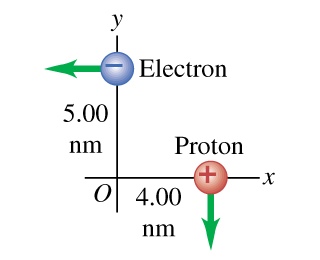# Problem: An electron and a proton are each moving at 760 km/s in perpendicular paths as shown in .1. At the instant when they are at the positions shown, find the magnitude of the total magnetic field they produce at the origin.2. Find the magnitude of the magnetic field the electron produces at the location of the proton.3. Find the magnitude of the total magnetic force that the electron exerts on the proton.4. Find the direction of the total magnetic field they produce at the origin.5. Find the direction of the magnetic field the electron produces at the location of the proton.6. Find the direction of the total magnetic force that the electron exerts on the proton.7. Find the magnitude of the total electrical force that the electron exerts on the proton.8. Find the direction of the total electrical force that the electron exerts on the proton.

###### FREE Expert Solution

From Biot-Savart law, the magnitude of the magnetic field is:

$\overline{){\mathbf{B}}{\mathbf{=}}\frac{{\mathbf{\mu }}_{\mathbf{0}}\mathbf{q}\mathbf{v}\mathbf{s}\mathbf{i}\mathbf{n}\mathbf{\theta }}{\mathbf{4}\mathbf{\pi }{\mathbf{r}}^{\mathbf{2}}}}$###### Problem Details

An electron and a proton are each moving at 760 km/s in perpendicular paths as shown in.

1. At the instant when they are at the positions shown, find the magnitude of the total magnetic field they produce at the origin.

2. Find the magnitude of the magnetic field the electron produces at the location of the proton.

3. Find the magnitude of the total magnetic force that the electron exerts on the proton.

4. Find the direction of the total magnetic field they produce at the origin.

5. Find the direction of the magnetic field the electron produces at the location of the proton.

6. Find the direction of the total magnetic force that the electron exerts on the proton.

7. Find the magnitude of the total electrical force that the electron exerts on the proton.

8. Find the direction of the total electrical force that the electron exerts on the proton.